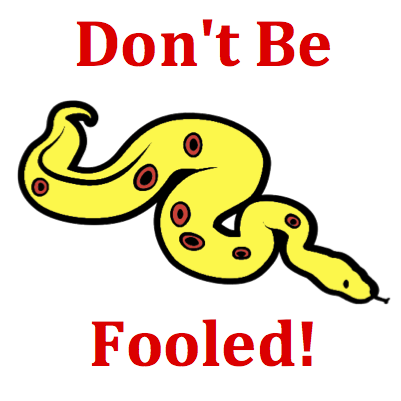# Kinematic Graphing - Mission KG6 Detailed HelpThe motion of an object is depicted by a velocity-time graph. Various time intervals during the motion are depicted by a letter. During which time interval(s) is the object speeding up (getting faster)? List all that apply ... .Velocity-Time Graphs: Velocity versus time graphs represent changes that occur in an object's velocity with respect to time. The slope of the line is the acceleration (change in velocity divided by the change in time) of the object. The area under the line (between the line on the graph and the time axis) is the displacement of the object.Many students become confused by the phrase speeding up. Because of the presence of up in the phrase, they begin to restrict their answers to those lines which are sloping up. But don't be fooled! Sloping up does not mean speeding up. A line which simply slopes up is a line with a positive slope and thus a positive acceleration. An object which speeds up is an object with an increasing speed. An object can move in the negative direction (and have a line sloping downward) and still be speeding up (if the line is getting further from the time axis). See Think About It section.Many questions in this mission can be answered with a mixture of good math sense and logical thinking. The graph is a plot of velocity. Velocity is a speed with a direction. Since the magnitude of the velocity is the speed, speeding up means getting bigger velocity values. The horizontal axis on the graph represents a 0 velocity. As a line on the graph gets further away from this 0 value, the velocity value is increasing. Whether the line goes up and away from the axis or down and away from the axis, the velocity values are getting bigger. A line going down and away from the time axis means that the velocity is attaining bigger negative values. This means that the object's speed is increasing as the object moves in the negative direction.